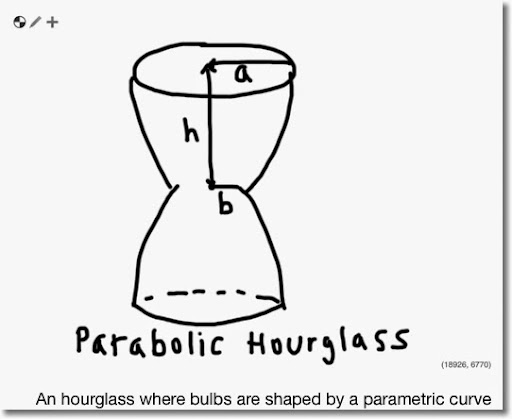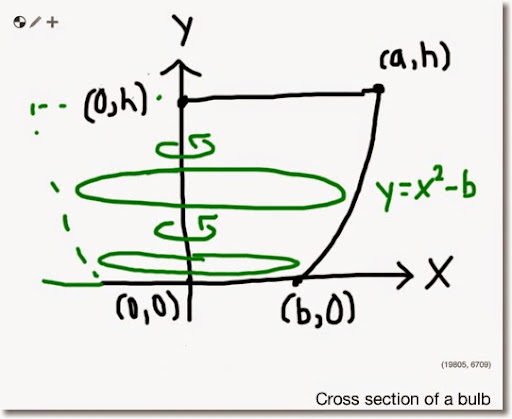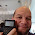## Tuesday, July 1, 2014

### Parabolic HourglassLet the above picture represent a "parabolic hourglass", where the two bulbs are outlined by a parabolic curve. Assume that each of the bulb is equal in size.

To calculate the volume of the parabolic hourglass, first calculate the volume of one of the two bulbs. The total volume of the parabolic hourglass is twice the volume of a bulb.

Examining one of the bulbs, suppose the edge of the bulb can be described by the equation y = x^2 - b. See the diagram below, where we impose a cross section of the bulb on the Cartesian plane.Note that:

(1) The small art of the bulb is placed on the x-axis, and

(2) The origin (point (0,0)) is placed in the center of the base.

To calculate the volume, we are going to use the Disc Integration Method with the discs rotating around the y-axis. The general formula for this method with y-axis
(x = 0) as the axis of rotation is:

d
∫ (r(y))^2 dy * π
c

We have the following constraints:

Upper: y = h
Lower: y = 0
Left: x = 0
Right: x = √(y + b)

And r(y) = √(y + b) - 0 = √(y + b)

With c = 0 and d = h, the volume of one bulb is:

h
∫ (√(y + b))^2 dy * π
0

h
∫ y + b dy * π
0

h
[ y^2/2 + b*y ] * π
0

π * (h^2/2 + b*h) (I)

If we want to determine the volume of the bulb in terms of a (outer radius), use the equation y = x^2 + b and the point (a, h) to determine that:

h = a^2 - b

b = a^2 - h (II)

Substitute equation (II) into (I) to get:

π * (h^2/2 + a^2 * h - h^2)

π * (a^2 * h - h^2/2) (III)

Remember that this the volume of one bulb. The parabolic hourglass consists of two equally sized bulbs.

Therefor the volume of the parabolic hourglass is:

V = 2 * π * (h^2/2 + b * h) = 2 * π * (a^2 * h - h^2/2)

Eddie

This blog is property of Edward Shore. 2014

#### 5 comments:

1.Fantastic topic and great set of instructions. Thanks Eddie!

2.This comment has been removed by the author.

3.Have a related question for you. Will be great if you solved it for me. What would be the shape of the hourglass so that the radius of the brim reduces at a constant rate, assuming constant volume leakage?

1.Kaustav,

Sorry for the late reply.

Let A be the radius of the brim at time t. Since the radius of the brim radius decreases at a constant rate, we have:

dA/dt = -c

Taking the integral, we get:

A = -c*t + K

Let a be the original radius of the brim. Hence, A(0) = a, and:

a = -c*0 + K
K = 0, which leads to:

A(t) = -c*t + a

We can calculate the volume at any time t at:

V = 2 * π * (A^2 * h - h^2/2)

where A = -c*t + a

Eddie

4.Nice Post thanks
consumerreports login

### Swiss Micros DM16L: Advanced Boolean and Factorial (up to 20)

Swiss Micros DM16L:   Advanced Boolean and Factorial (up to 20) Introduction The program listing, for the Swiss Micros DM16L and Hewlett Pac...